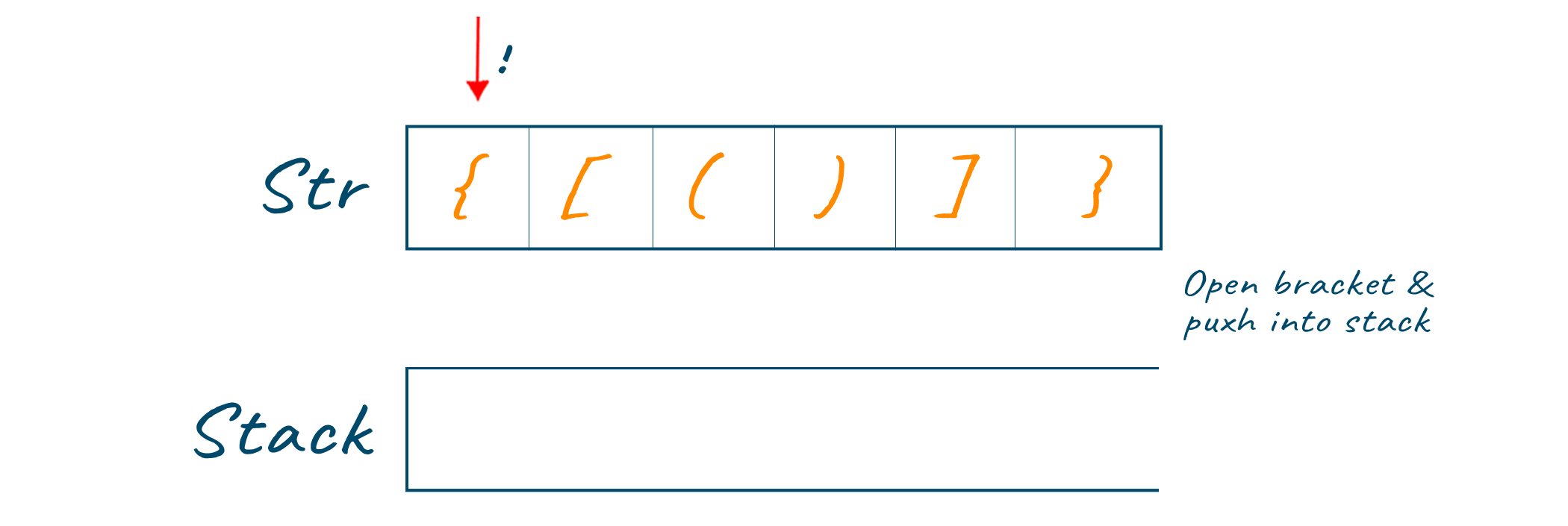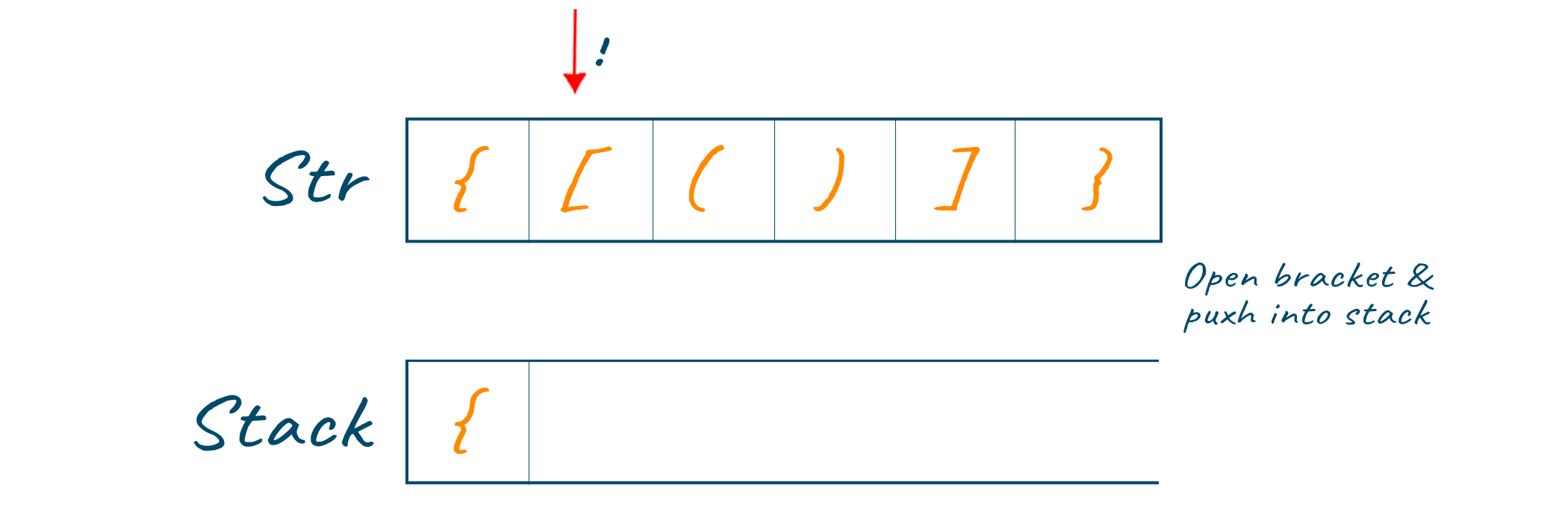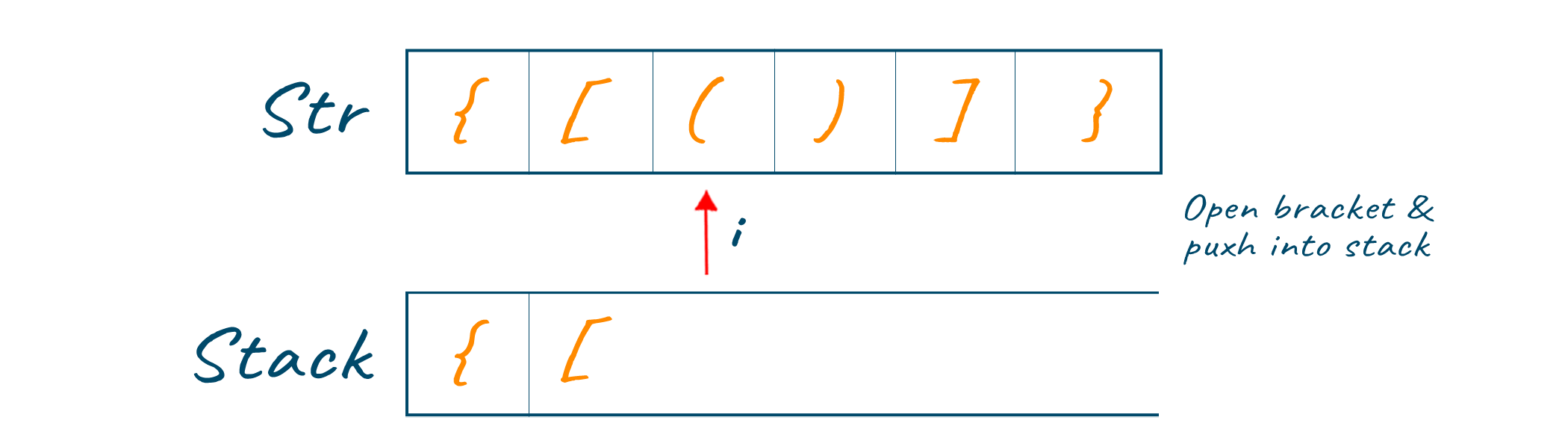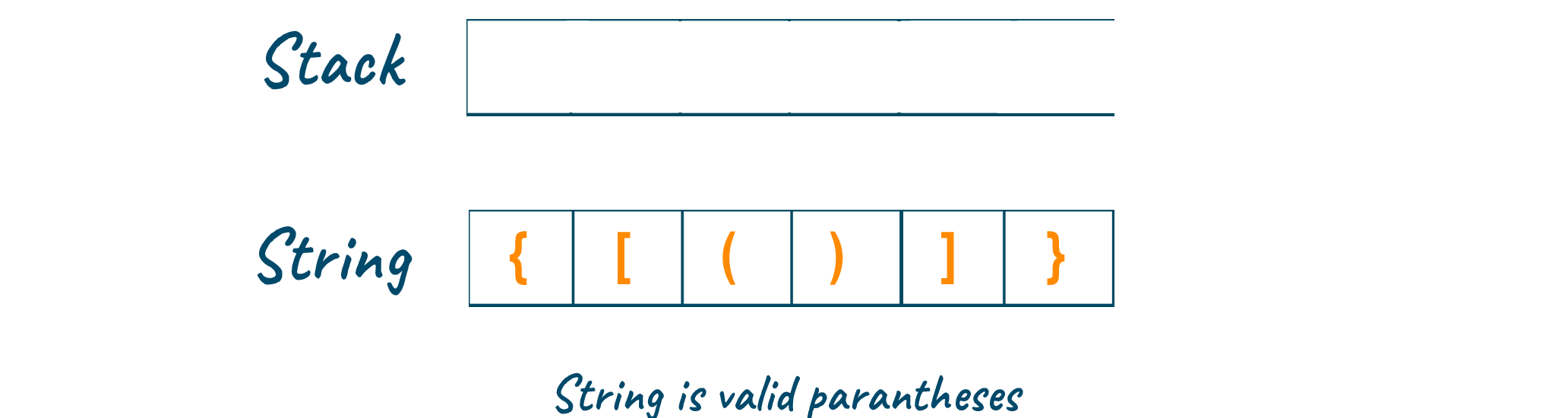# Check for Valid Balanced Parentheses in Python (with code)

Valid Parentheses (or Balanced Parentheses) play a crucial role while dealing with any programming language and hence, a programmer must understand it completely. Checking the valid parentheses in an expression is one of the commonly asked questions during technical interviews. So, to help you get the job in your dream company, let us discuss solutions using different approaches in Python.

But before that, let us understand the valid parentheses problem below.

## What is the Valid Parentheses Problem?

Parentheses are said to be balanced if each left parenthesis has its respective right parenthesis to match its pair in a well-nested format. In computer science, valid parentheses are a notation used to simplify expressions written in an operator-precedence parser by allowing the programmer to explicitly mark the operator precedence and associativity of a given expression.

Here is the problem statement: Given a string s containing just the characters '(', ')', '{', '}', '[', and ']', determine if the input string is valid or not.

Note that an input string is valid if:

• Open brackets must be closed by the same type of brackets
• Open brackets must be closed in the correct order

Now that you understand the problem statement correctly let us jump to understanding the solution of the problem in detail below.

## Algorithm for Valid Parenthesis Solution

Checking if a string of brackets is "valid" is a typical coding challenge known as the valid brackets problem. A string of parentheses is considered legitimate if each opening parenthesis is followed by a matching closing parenthesis in the appropriate placement.

The valid brackets problem can be solved using the following algorithm:

1. Make a stack that is empty.
2. Go through each character in the input string iteratively.
3. Push the character onto the stack if it is an opening parenthesis, such as "(," "," or "[."
4. Pop the top element from the stack if the current character is a closing parenthesis (such as ')', '', or ']'.
5. The string is invalid if the element that pops up is not the proper opening parenthesis.
6. The string is legitimate if the stack is empty after iterating over all of the characters in the string.

The Python code for this algorithm is provided here:

```def is_valid_parentheses(s):
stack = []
mapping = {")": "(", "}": "{", "]": "["}
for char in s:
if char in mapping:
top_element = stack.pop() if stack else '#'
if mapping[char] != top_element:
return False
else:
stack.append(char)
return not stack
```

An empty stack and a mapping from closing brackets to corresponding opening brackets are the first things we define in this code. The algorithm's steps are then carried out iteratively, one step per character in the input string.

The function returns True if the string is legitimate. Otherwise, False is returned.

## How to Check Balanced Parentheses in Expression?

Below are 3 methods by which you can check for Balanced parentheses in expression:

### 1) Using the Brute Force approach

The first thing that comes to your mind while solving the valid parentheses problem is by using the brute force approach. In this approach, you can make use of recursion, i.e., conditional statements and loop to match the parentheses, and if it returns true, the parentheses are valid; otherwise, not.

Here we make use of the while loop to check the sequence of parentheses and return the output of whether the parentheses are valid or not.

Check out the below Python code for checking valid parentheses using the brute force approach:

```class Solution:
def isValid( self, sequence ):
'''
Function to check if sequence contains valid parenthesis
:param sequence: Sequence of brackets
:return: True is sequence is valid else False
'''
# Replace the proper pairs until sequence becomes empty or no pairs are present
while True:
if '()' in sequence :
sequence = sequence.replace ( '()' , '' )
elif '{}' in sequence :
sequence = sequence.replace ( '{}' , '' )
elif '[]' in sequence :
sequence = sequence.replace ( '[]' , '' )
else :
return not sequence

if __name__ == '__main__':
sequence = '{[()]}'
print(f'Is {sequence} valid ? : {Solution().isValid(sequence)}')
sequence1 = '{[()]}{]{}}'
print(f'Is {sequence1} valid ? : {Solution().isValid (sequence1)}')```

Output:

```Is {[()]} valid ? : True
Is {[()]}{]{}} valid ? : False```

### 2) Checking Valid Parentheses using Stack

To solve a valid parentheses problem optimally, you can make use of Stack data structure.

Here you traverse through the expression and push the characters one by one inside the stack. Later, if the character encountered is the closing bracket, pop it from the stack and match it with the starting bracket. This way, you can check if the parentheses find their respective pair at the end of the traversal and if not, the parentheses are not valid.

Here is the algorithm for this optimal approach:

1. 1Declare stack S.
2. Now traverse the string expression using a pointer.
• if the current pointer is at the opening bracket ('(' or '{' or '[') then push it to stack S.
• else the current pointer is at closing bracket (')' or '}' or ']') then pop from the stack
• if the popped bracket is the matching opening bracket then brackets are valid
• else brackets are not valid.

After complete traversal, if there is some starting bracket left in the stack then "not valid"

Here is the code to check for balanced parentheses using stack in Python:

```class Solution:
def isValid (self, sequence: str):
'''
Function to pair if sequence contains valid parenthesis
:param sequence: Sequence of brackets
:return: True is sequence is valid else False
'''
stack = []
opening = set('([{')
closing = set(')]}')
pair = {')' : '(' , ']' : '[' , '}' : '{'}
for i in sequence :
if i in opening :
stack.append(i)
if i in closing :
if not stack :
return False
elif stack.pop() != pair[i] :
return False
else :
continue
if not stack :
return True
else :
return False

if __name__ == '__main__':
sequence = '{[()]}'
print(f'Is {sequence} valid ? : {Solution().isValid(sequence)}')
sequence1 = '{[()]}{]{}}'
print(f'Is {sequence1} valid ? : {Solution().isValid (sequence1)}')
```

Output:

```Is {[()]} valid ? : True
Is {[()]}{]{}} valid ? : False
```

The time complexity to check the valid parentheses using the optimal solution is O(n), where 'n' is the total number of characters in the expression. It is because we traverse through the sequence only once, and hence, the time complexity is linear in nature.

Similarly, the space complexity for this solution is O(n) as we require the stack of size 'n' to fit the characters of the expression.

To better understand it, let us consider the below expression for understanding the solution of the valid parentheses problem using stack.Initially, we will start traversing through the expression and push the open brackets inside the stack data structure, as shown in the below image.Keep repeating the same process until all the opening brackets are pushed inside the stack, and the pointer reaches forward.As soon as the pointer reaches the closing brackets, check the top of the stack if the respective opening bracket is the same kind or not. Pop the opening bracket from the stack for matching the pair of parenthesis as shown in the below image.Keep repeating the same process until all the closing brackets are visited by the pointer as shown in the below image.At last, if there is any bracket left inside the stack, it means that there is one matching pair of parenthesis left and the expression does not contain the valid parentheses. If all the brackets are popped out of the stack, it means the expression contains valid parentheses, as shown in the below image.### 3) Valid Parenthesis Solution without Stack

The valid brackets issue can also be resolved without the aid of a stack data structure. One method is to count the number of opening and closing brackets using a counter. The string is known to be invalid if the counter ever turns negative. We check the counter at the conclusion of each loop to see if the string is still valid.

The Python code to resolve the valid parentheses issue without utilizing a stack is shown below:

```def is_valid(s: str) -> bool:
count = 0
for c in s:
if c == "(":
count += 1
elif c == ")":
count -= 1
if count < 0:
return False
return count == 0
```

When a string s is passed to the is_valid function, it will either return the boolean value True or False, depending on whether or not the text has a valid set of brackets.

In order to count the opening and closing brackets in the string, the function initializes a counter variable count to zero. The function then employs a for loop to iterate through each character in the input string, s.

## Conclusion

Brackets play a primary role while programming in any language, especially in array indexing. They are also used in general denotation for tuples, sets, and many other data structures. In this article, we studied different approaches to check whether the expression is valid parentheses or not along with implementation in Python.

### FavTutor - 24x7 Live Coding Help from Expert Tutors!